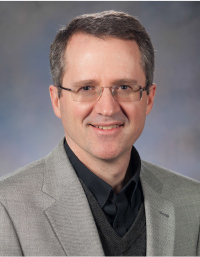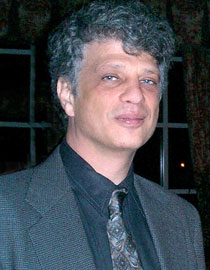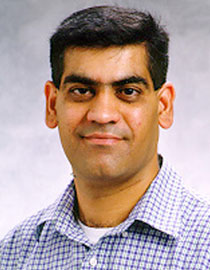## Research Faculty

### Tim Davis

• Professor, Computer Science & Engineering### Lawrence Rauchwerger

• Emeritus Professor, Computer Science & Engineering### Vivek Sarin

• Associate Professor, Computer Science & Engineering### Nate Veldt

• Assistant Professor, Computer Science & Engineering### Courses Offered

#### CSCE 653. Computer Methods in Applied Sciences. Credits 3. 3 Lecture Hours

Classical and modern techniques for the computational solution of problems of the type that traditionally arise in the natural sciences and engineering; introductions to number representation and errors, locating roots of equations, interpolation, numerical integration, linear algebraic systems, spline approximations, initial-value problems for ordinary differential equations and finite-difference methods for partial differential equations.

Prerequisite: CSCE 442 or MATH 417; graduate classification.

#### CSCE 657/PETE 657. High Performance Computing For Earth Science and Petroleum Engineering. Credits 3. 3 Lecture Hours

Numerical simulation of problems in Earth Sciences and Petroleum Engineering using high performance computing (HPC); development of a parallel reservoir simulator.

Cross Listing: PETE 657/CSCE 657.

#### CSCE 659/ECEN 659. Parallel/Distributed Numerical Algorithms and Applications. Credits 3. 3 Lecture Hours

A unified treatment of parallel and distributed numerical algorithms; parallel and distributed computation models, parallel computation of arithmetic expressions; fast algorithms for numerical linear algebra, partial differential equations and nonlinear optimization.

Prerequisite: CSCE 653MATH 304.
Cross Listing: ECEN 659/CSCE 659.

#### CSCE 660/MATH 660. Computational Linear Algebra. Credits 3. 3 Lecture Hours

Techniques in matrix computation: elimination methods, matrix decomposition, generalized inverses, orthogonalization and least-squares, eigenvalue problems and singular value decomposition, iterative methods and error analysis.

Prerequisite: CSCE 442 or equivalent or MATH 417 or equivalent.
Cross Listing: MATH 660/CSCE 660.